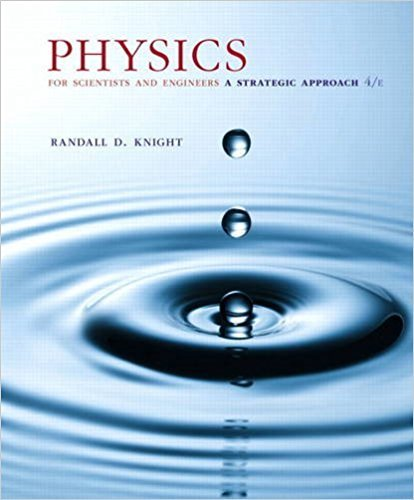×
×

# Consider a lens having index of refraction n2 and surfacesISBN: 9780134081496 191

## Solution for problem 34.118 Chapter 34

Physics for Scientists and Engineers: A Strategic Approach, Standard Edition (Chs 1-36) | 4th Edition

• Textbook Solutions
• 2901 Step-by-step solutions solved by professors and subject experts
• Get 24/7 help from StudySoup virtual teaching assistantsPhysics for Scientists and Engineers: A Strategic Approach, Standard Edition (Chs 1-36) | 4th Edition

4 5 0 315 Reviews
10
4
Problem 34.118

Consider a lens having index of refraction n2 and surfaces with radii R1 and R2. The lens is immersed in a fluid that has index of refraction n1. a. Derive a generalized lens makers equation to replace Equation 34.26 when the lens is surrounded by a medium other than air. That is, when n1 1. b. A symmetric converging glass lens (i.e., two equally curved surfaces) has two surfaces with radii of 40 cm. Find the focal length of this lens in air and the focal length of this lens in water

Step-by-Step Solution:
Step 1 of 3

Technology of Alpine Skiing ME1520 Homework 1. Time of descent and terminal velocity Consider a 90kg skier with a coefficient of drag of 0.5, frontal area of 0.4 m², when the air density is 1.2 kg/m³, and the ski-snow friction coefficient is 0.03, with an initial speed of from an initial speed of 2m/s at point A 1 (path ABC) On a slope that is initially 30deg. a skier...

Step 2 of 3

Step 3 of 3

##### ISBN: 9780134081496

Since the solution to 34.118 from 34 chapter was answered, more than 227 students have viewed the full step-by-step answer. The answer to “Consider a lens having index of refraction n2 and surfaces with radii R1 and R2. The lens is immersed in a fluid that has index of refraction n1. a. Derive a generalized lens makers equation to replace Equation 34.26 when the lens is surrounded by a medium other than air. That is, when n1 1. b. A symmetric converging glass lens (i.e., two equally curved surfaces) has two surfaces with radii of 40 cm. Find the focal length of this lens in air and the focal length of this lens in water” is broken down into a number of easy to follow steps, and 92 words. The full step-by-step solution to problem: 34.118 from chapter: 34 was answered by , our top Physics solution expert on 12/28/17, 08:06PM. This full solution covers the following key subjects: . This expansive textbook survival guide covers 42 chapters, and 4463 solutions. This textbook survival guide was created for the textbook: Physics for Scientists and Engineers: A Strategic Approach, Standard Edition (Chs 1-36), edition: 4. Physics for Scientists and Engineers: A Strategic Approach, Standard Edition (Chs 1-36) was written by and is associated to the ISBN: 9780134081496.

Unlock Textbook Solution